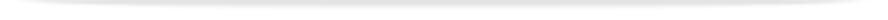units

index-byarea of study-m-mathematics and statistics

# Units indexed by Area of study: Mathematics and statistics

## Other areas of study

For detailed information about the sequence of units required for a major/minor/specialisation in this area of study refer to Mathematics and statistics

• ASP2062 Introduction to astrophysics
• ASP3012 Stars and galaxies
• ASP3051 Relativity and cosmology
• ATM2020 Climate dynamics of the atmosphere and oceans
• ATM2030 Clouds, weather and forecasting
• ATM3040 Physical meteorology
• ATM3050 Dynamical meteorology
• MAT1830 Discrete mathematics for computer science
• MTH1000 Special topics in mathematics level one
• MTH1010 Functions and their applications
• MTH1020 Analysis of change
• MTH1030 Techniques for modelling
• MTH1035 Techniques for modelling (advanced)
• MTH2000 Mathematics research project level 2
• MTH2010 Multivariable calculus
• MTH2021 Linear algebra with applications
• MTH2032 Differential equations with modelling
• MTH2121 Algebra and number theory
• MTH2132 The nature and beauty of mathematics
• MTH2140 Real analysis
• MTH2222 Mathematics of uncertainty
• MTH2232 Mathematical statistics
• MTH3000 Mathematics research project level 3
• MTH3011 Partial differential equations
• MTH3020 Complex analysis and integral transforms
• MTH3051 Introduction to computational mathematics
• MTH3060 Advanced ordinary differential equations
• MTH3110 Differential geometry
• MTH3121 Algebra and number theory
• MTH3140 Real analysis
• MTH3150 Algebra and number theory II
• MTH3160 Functional analysis
• MTH3230 Time series and random processes in linear systems
• MTH3241 Random processes in the sciences and engineering
• MTH3251 Financial mathematics
• MTH3310 Applied mathematical modelling
• MTH3360 Fluid dynamics
• STA1010 Statistical methods for science